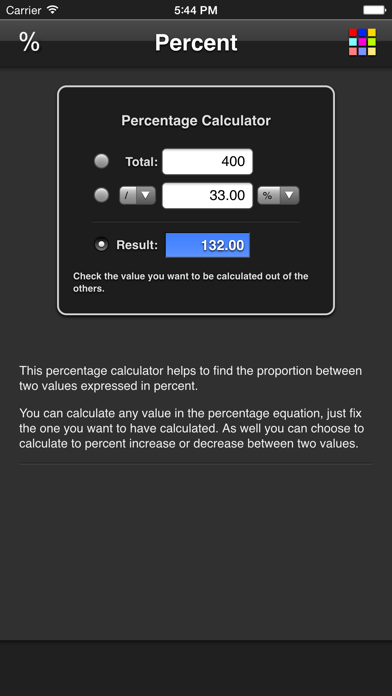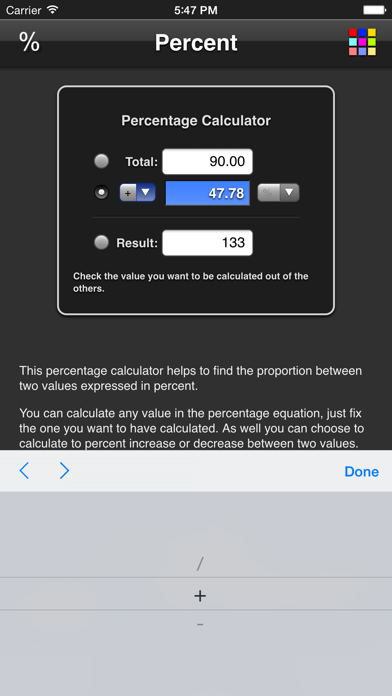# Percentage

## iOS UniversalUtilities

\$0.99

This percentage calculator helps to find the proportion between two values expressed in percent.

You can calculate any value in the percentage equation, just fix the one you want to have calculated. You can choose to calculate the percent increase or decrease between two values as well.

## What's New

- support for iPhone X+

•••••